CAT  >  Practice Questions Level 1: Missing Data

# Practice Questions Level 1: Missing Data - Notes | Study Level-wise Practice Questions for CAT Preparation - CAT

 1 Crore+ students have signed up on EduRev. Have you?

This EduRev document offers 15 Multiple Choice Questions (MCQs) from the topic Missing Data (Level - 1). These questions are of Level - 1 difficulty and will assist you in the preparation of CAT & other MBA exams. You can practice/attempt these CAT Multiple Choice Questions (MCQs) and check the explanations for a better understanding of the topic.

Question for Practice Questions Level 1: Missing Data
Try yourself:Directions: Study the following information and answer the question that follows.

The table below shows the percentage profit or loss of five different commodities based on the sum of cost price and shipping cost.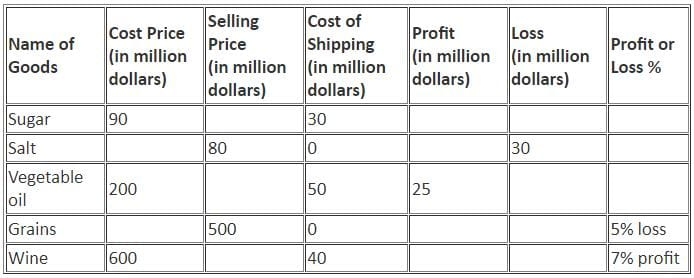Question for Practice Questions Level 1: Missing Data
Try yourself:Directions: Study the following information and answer the question that follows.

The table below shows the percentage profit or loss of five different commodities based on the sum of cost price and shipping cost.Q. What is the difference between the selling price of Wine and that of Vegetable oil?

Question for Practice Questions Level 1: Missing Data
Try yourself:Directions: Study the following information and answer the question that follows.

The table below shows the percentage profit or loss of five different commodities based on the sum of cost price and shipping cost.Q. What is the ratio of the loss on Grains to that on Salt?

Question for Practice Questions Level 1: Missing Data
Try yourself:Directions: Study the given information and answer the following question.
The table given below shows the estimated cost (in Rs. lakh) of a project of laying a railway line between two places, the project began in 1988 and was completed in 1991.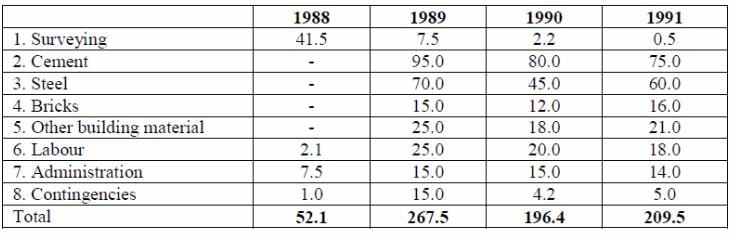Q. How many times approximately was the total cost of materials to the total labour cost for all the years?

Question for Practice Questions Level 1: Missing Data
Try yourself:Directions: Study the given information and answer the following question.
The table given below shows the estimated cost (in Rs. lakh) of a project of laying a railway line between two places, the project began in 1988 and was completed in 1991.Q. The total expenditure was required to be kept within Rs. 700 lakh by cutting the expenditure on administration equally in all the years. What was the percentage cut on administration expenditure for the year 1989?

Question for Practice Questions Level 1: Missing Data
Try yourself:Directions: Study the given information and answer the following question.
The table given below shows the estimated cost (in Rs. lakh) of a project of laying a railway line between two places, the project began in 1988 and was completed in 1991.Q. If the length of line to be laid each year was in proportion to the estimated cost for material and labour, what fraction of the total length was proposed to be completed by the third year?

Question for Practice Questions Level 1: Missing Data
Try yourself:Directions: Study the following information carefully and answer the question given below.
Table shows income, increment percentage, expenses and saving details of three employees for three years (2015, 2016 and 2017).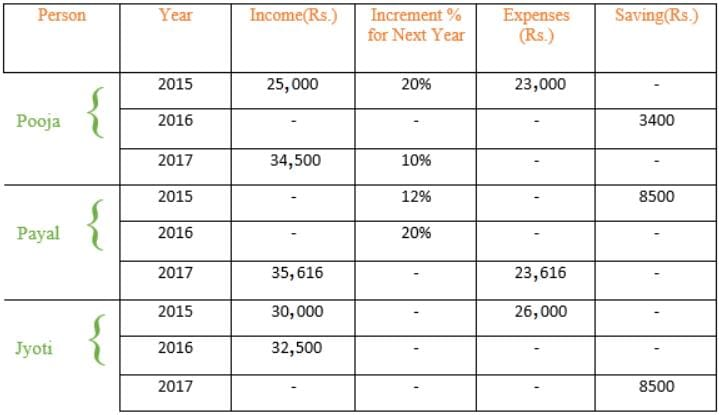Q. If the difference between the expenses and savings of Jyoti in 2017 is Rs. 16,750, then what is the increment percentage in the total income of Jyoti from 2015 to 2017?

Question for Practice Questions Level 1: Missing Data
Try yourself:Directions: Study the following information carefully and answer the question given below.
Table shows income, increment percentage, expenses and saving details of three employees for three years (2015, 2016 and 2017).Q. If the income of Pooja in 2015 is doubled and the percentage increment of Pooja in the year 2016 is also doubled, then what is the income of Pooja in 2017?

Question for Practice Questions Level 1: Missing Data
Try yourself:Directions: Study the following information carefully and answer the question given below.
Table shows income, increment percentage, expenses and saving details of three employees for three years (2015, 2016 and 2017).Q. Income of Pooja in 2016 is approximately what percentage more/less than the average income of Pooja?

Question for Practice Questions Level 1: Missing Data
Try yourself:Directions: Study the following information carefully and answer the question given below.
Table shows income, increment percentage, expenses and saving details of three employees for three years (2015, 2016 and 2017).Q. If the income of Pooja in 2015 is doubled and the percentage increment of Pooja in the year 2016 is also doubled, then what is the income of Pooja in 2017?

Question for Practice Questions Level 1: Missing Data
Try yourself:Directions: Study the table given below and answer the following question.
Distribution of washing machines in different cities and among different categories of people of Telangana is as follows:
(Total washing machines distributed = 30 lakh)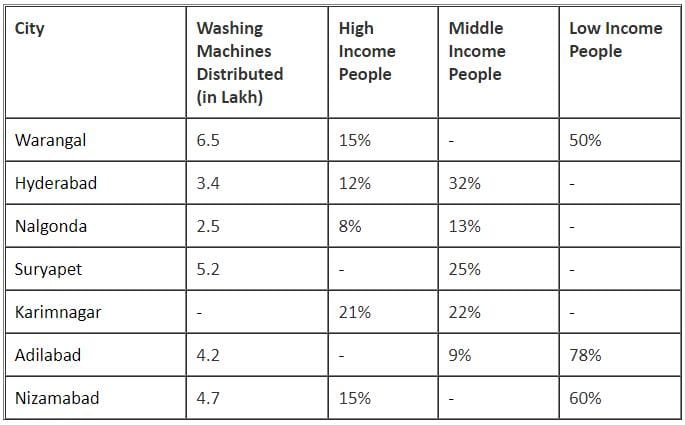Q. What is the difference between total distribution of washing machines in Warangal and total distribution of washing machines in Karimnagar and Adilabad together?

Question for Practice Questions Level 1: Missing Data
Try yourself:Directions: Study the table given below and answer the following question.
Distribution of washing machines in different cities and among different categories of people of Telangana is as follows:
(Total washing machines distributed = 30 lakh)Q. In Suryapet city, the ratio of percent distribution of Washing Machines among high income people to that among low income people is 2 : 3. Then, how much more is the distribution of Washing Machines among high income people in the same city than that among middle income people?

Question for Practice Questions Level 1: Missing Data
Try yourself:Directions: Study the table given below and answer the following question.
Distribution of washing machines in different cities and among different categories of people of Telangana is as follows:
(Total washing machines distributed = 30 lakh)Q. What is the difference between the number of Washing Machines distributed among high income people of Nalgonda, Warangal and Nizamabad together and that distributed among middle income people of Suryapet, Karimnagar and Nizamabad together?

Question for Practice Questions Level 1: Missing Data
Try yourself:Directions: Study the following graph carefully and answer the question that follows: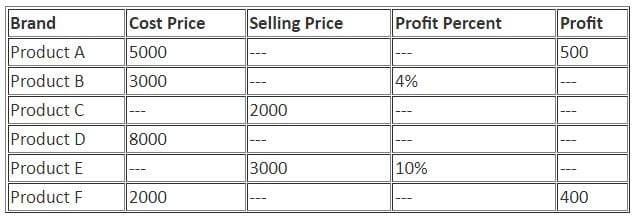Q. What is the profit or loss % of product C if cost price of product C is half the cost price of product D?

Question for Practice Questions Level 1: Missing Data
Try yourself:Directions: Study the following graph carefully and answer the question that follows:Q. What is the profit of product B?

The document Practice Questions Level 1: Missing Data - Notes | Study Level-wise Practice Questions for CAT Preparation - CAT is a part of the CAT Course Level-wise Practice Questions for CAT Preparation.
All you need of CAT at this link: CAT

## Level-wise Practice Questions for CAT Preparation

277 docs
 Use Code STAYHOME200 and get INR 200 additional OFF

## Level-wise Practice Questions for CAT Preparation

277 docs

### How to Prepare for CAT

Read our guide to prepare for CAT which is created by Toppers & the best Teachers

Track your progress, build streaks, highlight & save important lessons and more!

,

,

,

,

,

,

,

,

,

,

,

,

,

,

,

,

,

,

,

,

,

;